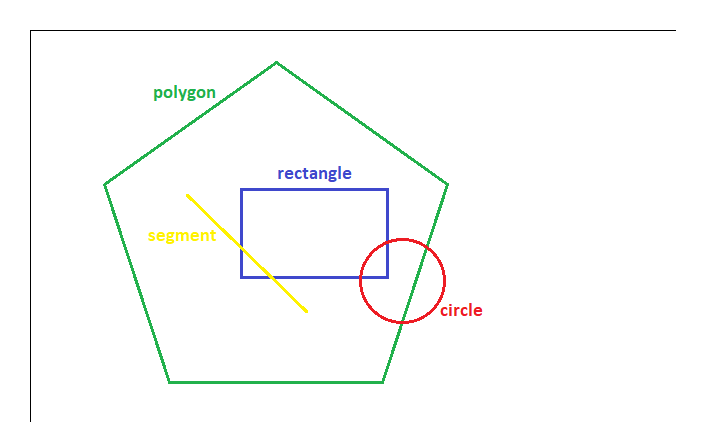npm's personal messenger

# npm

## shapes-interaction

1.0.4 • Public • Published

# Shapes-Interaction-Library

A lightweight library of basic geometry shapes interactions. Enables a possibility of shape intersection determination or situation when shape contains other shape.

## Installation

node :

web :

``````<script src="unpkg.com/shapes-interaction@1.0.4/index.min.js"></script>
``````

## API

This module exports two functions:

The `intersection` function takes two shapes as an input and returns 'true' if shapes intersect or 'false' if shapes do not intersect

`intersection(shape1, shape2)`

The `contain` function takes two shapes as an input and returns 'true' if first shape contains second shape or 'false' if first shape does not contain second shape

`contain(shape1, shape2)`

Functions maintain 5 types of shapes such as:

`point == [x, y]` (array of two coordinates of point)

`segment == [point, point] == [[x1, y1], [x2, y2]]` (array of two points)

`rectangle == [[point], width, height] == [[x, y], width, height]` (array of vertex, width and height of rectangle)

`circle == [[point], radius] == [[x, y], radius]` (array of centre and radius of circle)

`polygon == [[point1], [point2], ..., [pointN]] == [[x1, y1], [x2, y2], ..., [xN, yN]]` (array of N points)

## Usage example## Keywords

### Install

`npm i shapes-interaction`

6

1.0.4